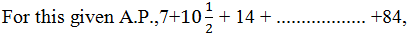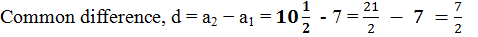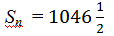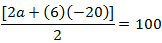NCERT Solutions for Class 10 Maths Chapter 5- Arithmetic Progressions Exercise 5.3 includes detailed answers to the problems provided in Exercise 5.3. These solutions are written to guide the students in the CBSE Class 10 second term examination.

In order to solve different types of questions on first n terms of arithmetic progressions, students are advised to study this NCERT solution thoroughly. By solving these exercise questions, you will be better equipped to comprehend all possible types of questions that may be asked in class 10 second term examinations.

## Topics covered in arithmetic progressions Exercise 5.3

1. Sum of First n Terms of an AP
2. Solved examples

• Helps you in comprehending the methodology to solve sum of first terms of an arithmetic progression
• Makes you memorise the important formulas and methodologies
• Assists you in scoring all the questions on arithmetic progression accurately

### Access other exercise solutions of Class 10 Maths Chapter 5- Arithmetic progression Exercise 5.3

1. Find the sum of the following APs.

(i) 2, 7, 12 ,…., to 10 terms.
(ii) − 37, − 33, − 29 ,…, to 12 terms
(iii) 0.6, 1.7, 2.8 ,…….., to 100 terms
(iv) 1/15, 1/12, 1/10, …… , to 11 terms

Solutions:

(i) Given, 2, 7, 12 ,…, to 10 terms

For this A.P.,

first term, a = 2

And common difference, d = a2 − a1 = 7−2 = 5

n = 10

We know that, the formula for sum of nth term in AP series is,

Sn = n/2 [2a +(n-1)d]

S10 = 10/2 [2(2)+(10 -1)×5]

= 5[4+(9)×(5)]

= 5 × 49 = 245

(ii) Given, −37, −33, −29 ,…, to 12 terms

For this A.P.,

first term, a = −37

And common difference, d = a2− a1

d= (−33)−(−37)

= − 33 + 37 = 4

n = 12

We know that, the formula for sum of nth term in AP series is,

Sn = n/2 [2a+(n-1)d]

S12 = 12/2 [2(-37)+(12-1)×4]

= 6[-74+11×4]

= 6[-74+44]

= 6(-30) = -180

(iii) Given, 0.6, 1.7, 2.8 ,…, to 100 terms

For this A.P.,

first term, a = 0.6

Common difference, d = a2 − a1 = 1.7 − 0.6 = 1.1

n = 100

We know that, the formula for sum of nth term in AP series is,

Sn = n/2[2a +(n-1)d]

S12 = 50/2 [1.2+(99)×1.1]

= 50[1.2+108.9]

= 50[110.1]

= 5505

(iv) Given, 1/15, 1/12, 1/10, …… , to 11 terms

For this A.P.,

First term, a = 1/5

Common difference, d = a–a1 = (1/12)-(1/5) = 1/60

And number of terms n = 11

We know that, the formula for sum of nth term in AP series is,

Sn = n/2 [2a + (n – 1) d]= 11/2(2/15 + 10/60)

= 11/2 (9/30)

= 33/20

2. Find the sums given below:(ii) 34 + 32 + 30 + ……….. + 10
(iii) − 5 + (− 8) + (− 11) + ………… + (− 230)

Solutions:

(i)First term, a = 7

nth term, a= 84Let 84 be the nth term of this A.P., then as per the nth term formula,

a= a(n-1)d

84 = 7+(n – 1)×7/2

77 = (n-1)×7/2

22 = n−1

n = 23

We know that, sum of n term is;

Sn = n/2 (a + l) , l = 84
Sn
= 23/2 (7+84)

Sn  = (23×91/2) = 2093/2(ii) Given, 34 + 32 + 30 + ……….. + 10

For this A.P.,

first term, a = 34

common difference, d = a2−a1 = 32−34 = −2

nth term, an= 10

Let 10 be the nth term of this A.P., therefore,

an= a +(n−1)d

10 = 34+(n−1)(−2)

−24 = (n −1)(−2)

12 = n −1

n = 13

We know that, sum of n terms is;

Sn = n/2 (a +l) , l = 10

= 13/2 (34 + 10)

= (13×44/2) = 13 × 22

= 286

(iii) Given, (−5) + (−8) + (−11) + ………… + (−230)

For this A.P.,

First term, a = −5

nth term, an= −230

Common difference, d = a2−a1 = (−8)−(−5)

⇒d = − 8+5 = −3

Let −230 be the nth term of this A.P., and by the nth term formula we know,

ana+(n−1)d

−230 = − 5+(n−1)(−3)

−225 = (n−1)(−3)

(n−1) = 75

n = 76

And, Sum of n term,

Sn = n/2 (a + l)

= 76/2 [(-5) + (-230)]

= 38(-235)

= -8930

3. In an AP
(i) Given a = 5, d = 3, an = 50, find n and Sn.
(ii) Given a = 7, a13 = 35, find d and S13.
(iii) Given a12 = 37, d = 3, find a and S12.
(iv) Given a3 = 15, S10 = 125, find d and a10.
(v) Given d = 5, S9 = 75, find a and a9.
(vi) Given a = 2, d = 8, Sn = 90, find n and an.
(vii) Given a = 8, an = 62, Sn = 210, find n and d.
(viii) Given an = 4, d = 2, Sn = − 14, find n and a.
(ix) Given a = 3, n = 8, S = 192, find d.
(x) Given l = 28, S = 144 and there are total 9 terms. Find a.

Solutions:

(i) Given that, a = 5, d = 3, an = 50

As we know, from the formula of the nth term in an AP,

an = a +(n −1)d,

Therefore, putting the given values, we get,

⇒ 50 = 5+(n -1)×3

⇒ 3(n -1) = 45

⇒ n -1 = 15

⇒ n = 16

Now, sum of n terms,

Sn = n/2 (a +an)

Sn = 16/2 (5 + 50) = 440

(ii) Given that, a = 7, a13 = 35

As we know, from the formula of the nth term in an AP,

an = a+(n−1)d,

Therefore, putting the given values, we get,

⇒ 35 = 7+(13-1)d

⇒ 12d = 28

⇒ d = 28/12 = 2.33

Now, Sn = n/2 (a+an)

S13 = 13/2 (7+35) = 273

(iii) Given that, a12 = 37, d = 3

As we know, from the formula of the nth term in an AP,

an = a+(n −1)d,

Therefore, putting the given values, we get,

⇒ a12 = a+(12−1)3

⇒ 37 = a+33

⇒ a = 4

Now, sum of nth term,

Sn = n/2 (a+an)

Sn = 12/2 (4+37)

= 246

(iv) Given that, a3 = 15, S10 = 125

As we know, from the formula of the nth term in an AP,

an = a +(n−1)d,

Therefore, putting the given values, we get,

a3 = a+(3−1)d

15 = a+2d ………………………….. (i)

Sum of the nth term,

Sn = n/2 [2a+(n-1)d]

S10 = 10/2 [2a+(10-1)d]

125 = 5(2a+9d)

25 = 2a+9……………………….. (ii)

On multiplying equation (i) by (ii), we will get;

30 = 2a+4d ………………………………. (iii)

By subtracting equation (iii) from (ii), we get,

−5 = 5d

d = −1

From equation (i),

15 = a+2(−1)

15 = a−2

a = 17 = First term

a10 = a+(10−1)d

a10 = 17+(9)(−1)

a10 = 17−9 = 8

(v) Given that, d = 5, S9 = 75

As, sum of n terms in AP is,

Sn = n/2 [2a +(n -1)d]

Therefore, the sum of first nine terms are;

S9 = 9/2 [2a +(9-1)5]

25 = 3(a+20)

25 = 3a+60

3a = 25−60

a = -35/3

As we know, the nth term can be written as;

an = a+(n−1)d

a9 = a+(9−1)(5)

= -35/3+8(5)

= -35/3+40

= (35+120/3) = 85/3

(vi) Given that, a = 2, d = 8, Sn = 90

As, sum of n terms in an AP is,

Sn = n/2 [2a +(n -1)d]

90 = n/2 [2a +(n -1)d]

⇒ 180 = n(4+8n -8) = n(8n-4) = 8n2-4n

⇒ 8n2-4n –180 = 0

⇒ 2n2n-45 = 0

⇒ 2n2-10n+9n-45 = 0

⇒ 2n(n -5)+9(n -5) = 0

⇒ (n-5)(2n+9) = 0

So, n = 5 (as n only be a positive integer)

∴ a5 = 8+5×4 = 34

(vii) Given that, a = 8, an = 62, Sn = 210

As, sum of n terms in an AP is,

Sn = n/2 (a + an)

210 = n/2 (8 +62)

⇒ 35n = 210

⇒ n = 210/35 = 6

Now, 62 = 8+5d

⇒ 5d = 62-8 = 54

⇒ d = 54/5 = 10.8

(viii) Given that, nth term, an = 4, common difference, d = 2, sum of n terms, Sn = −14.

As we know, from the formula of the nth term in an AP,

an = a+(n −1)d,

Therefore, putting the given values, we get,

4 = a+(−1)2

4 = a+2n−2

a+2n = 6

= 6 − 2n …………………………………………. (i)

As we know, the sum of n terms is;

Sn = n/2 (a+an)

-14 = n/2 (a+4)

−28 = (a+4)

−28 = (6 −2n +4) {From equation (i)}

−28 = (− 2n +10)

−28 = − 2n2+10n

2n2 −10n − 28 = 0

n2 −5−14 = 0

n2 −7n+2n −14 = 0

(n−7)+2(n −7) = 0

(n −7)(n +2) = 0

Either n − 7 = 0 or n + 2 = 0

n = 7 or n = −2

However, n can neither be negative nor fractional.

Therefore, n = 7

From equation (i), we get

a = 6−2n

a = 6−2(7)

= 6−14

= −8

(ix) Given that, first term, a = 3,

Number of terms, n = 8

And sum of n terms, S = 192

As we know,

Sn = n/2 [2a+(n -1)d]

192 = 8/2 [2×3+(8 -1)d]

192 = 4[6 +7d]

48 = 6+7d

42 = 7d

d = 6

(x) Given that, l = 28,S = 144 and there are total of 9 terms.

Sum of n terms formula,

Sn = n/2 (a + l)

144 = 9/2(a+28)

(16)×(2) = a+28

32 = a+28

a = 4

4. How many terms of the AP. 9, 17, 25 … must be taken to give a sum of 636?

Solutions:

Let there be n terms of the AP. 9, 17, 25 …

For this A.P.,

First term, a = 9

Common difference, d = a2a1 = 17−9 = 8

As, the sum of n terms, is;

Sn = n/2 [2a+(n -1)d]

636 = n/2 [2×a+(8-1)×8]

636 = n/2 [18+(n-1)×8]

636 = [9 +4n −4]

636 = (4n +5)

4n2 +5n −636 = 0

4n2 +53n −48n −636 = 0

(4n + 53)−12 (4n + 53) = 0

(4n +53)(n −12) = 0

Either 4n+53 = 0 or n−12 = 0

n = (-53/4) or n = 12

cannot be negative or fraction, therefore, n = 12 only.

5. The first term of an AP is 5, the last term is 45 and the sum is 400. Find the number of terms and the common difference.

Solution:

Given that,

first term, a = 5

last term, l = 45

Sum of the AP, Sn = 400

As we know, the sum of AP formula is;

Sn = n/2 (a+l)

400 = n/2(5+45)

400 = n/2(50)

Number of terms, n =16

As we know, the last term of AP series can be written as;

l = a+(n −1)d

45 = 5 +(16 −1)d

40 = 15d

Common difference, d = 40/15 = 8/3

6. The first and the last term of an AP are 17 and 350 respectively. If the common difference is 9, how many terms are there and what is their sum?

Solution:

Given that,

First term, a = 17

Last term, l = 350

Common difference, d = 9

Let there be n terms in the A.P., thus the formula for last term can be written as;

l = a+(n −1)d

350 = 17+(n −1)9

333 = (n−1)9

(n−1) = 37

n = 38

Sn = n/2 (a+l)

S38 = 38/2 (17+350)

= 19×367

= 6973

Thus, this A.P. contains 38 terms and the sum of the terms of this A.P. is 6973.

7. Find the sum of first 22 terms of an AP in which d = 7 and 22nd term is 149.
Solution:

Given,

Common difference, d = 7

22nd term, a22 = 149

Sum of first 22 term, S22 = ?

By the formula of nth term,

an = a+(n−1)d

a22 = a+(22−1)d

149 = a+21×7

149 = a+147

a = 2 = First term

Sum of n terms,

Sn = n/2(a+an)

S22 = 22/2 (2+149)

= 11×151

= 1661

8. Find the sum of first 51 terms of an AP whose second and third terms are 14 and 18 respectively.
Solution:

Given that,

Second term, a2 = 14

Third term, a3 = 18

Common difference, d = a3a2 = 18−14 = 4

a2 = a+d

14 = a+4

a = 10 = First term

Sum of n terms;

Sn = n/2 [2a + (n – 1)d]

S51 = 51/2 [2×10 (51-1) 4]

= 51/2 [20+(50)×4]

= 51 × 220/2

= 51 × 110

= 5610

9. If the sum of first 7 terms of an AP is 49 and that of 17 terms is 289, find the sum of first n terms.

Solution:

Given that,

S7 = 49

S17 = 289

We know, Sum of n terms;

Sn = n/2 [2a + (n – 1)d]

Therefore,

S77/2 [2a +(n -1)d]

S7 = 7/2 [2a + (7 -1)d]

49 = 7/2 [2a +6d]

7 = (a+3d)

a + 3d = 7 …………………………………. (i)

In the same way,

S17 = 17/2 [2a+(17-1)d]

289 = 17/2 (2a +16d)

17 = (a+8d)

a +8d = 17 ………………………………. (ii)

Subtracting equation (i) from equation (ii),

5d = 10

d = 2

From equation (i), we can write it as;

a+3(2) = 7

a+ 6 = 7

a = 1

Hence,

Sn = n/2[2a+(n-1)d]

n/2[2(1)+(n – 1)×2]

n/2(2+2n-2)

n/2(2n)

n2

10. Show that a1a… , an , … form an AP where an is defined as below

(i) an = 3+4n
(ii) an = 9−5n
Also find the sum of the first 15 terms in each case.

Solutions:

(i) an = 3+4n

a1 = 3+4(1) = 7

a2 = 3+4(2) = 3+8 = 11

a3 = 3+4(3) = 3+12 = 15

a4 = 3+4(4) = 3+16 = 19

We can see here, the common difference between the terms are;

a2 − a1 = 11−7 = 4

a3 − a2 = 15−11 = 4

a4 − a3 = 19−15 = 4

Hence, ak + 1 − ak is the same value every time. Therefore, this is an AP with common difference as 4 and first term as 7.

Now, we know, the sum of nth term is;

Sn = n/2[2a+(n -1)d]

S15 = 15/2[2(7)+(15-1)×4]

= 15/2[(14)+56]

= 15/2(70)

= 15×35

= 525

(ii) an = 9−5n

a1 = 9−5×1 = 9−5 = 4

a2 = 9−5×2 = 9−10 = −1

a3 = 9−5×3 = 9−15 = −6

a4 = 9−5×4 = 9−20 = −11

We can see here, the common difference between the terms are;

a2 − a1 = −1−4 = −5

a3 − a2 = −6−(−1) = −5

a4 − a3 = −11−(−6) = −5

Hence, ak + 1 − ak is same every time. Therefore, this is an A.P. with common difference as −5 and first term as 4.

Now, we know, the sum of nth term is;

Sn = n/2 [2a +(n-1)d]

S15 = 15/2[2(4) +(15 -1)(-5)]

= 15/2[8 +14(-5)]

= 15/2(8-70)

= 15/2(-62)

= 15(-31)

= -465

11. If the sum of the first n terms of an AP is 4n − n2, what is the first term (that is S1)? What is the sum of first two terms? What is the second term? Similarly find the 3rd, the10th and the nth terms.

Solution:

Given that,

Sn = 4nn2

First term, a = S1 = 4(1) − (1)2 = 4−1 = 3

Sum of first two terms = S2= 4(2)−(2)2 = 8−4 = 4

Second term, a2 = S2 − S1 = 4−3 = 1

Common difference, d = a2a = 1−3 = −2

Nth term, an = a+(n−1)

= 3+(n −1)(−2)

= 3−2n +2

= 5−2n

Therefore, a3 = 5−2(3) = 5-6 = −1

a10 = 5−2(10) = 5−20 = −15

Hence, the sum of first two terms is 4. The second term is 1.

The 3rd, the 10th, and the nth terms are −1, −15, and 5 − 2n respectively.

12. Find the sum of first 40 positive integers divisible by 6.

Solution:

The positive integers that are divisible by 6 are 6, 12, 18, 24 ….

We can see here, that this series forms an A.P. whose first term is 6 and common difference is 6.

a = 6

d = 6

S40 = ?

By the formula of sum of n terms, we know,

Sn = n/2 [2a +(n – 1)d]

Therefore, putting n = 40, we get,

S40 = 40/2 [2(6)+(40-1)6]

= 20[12+(39)(6)]

= 20(12+234)

= 20×246

= 4920

13. Find the sum of first 15 multiples of 8.

Solution:

The multiples of 8 are 8, 16, 24, 32…

The series is in the form of AP, having first term as 8 and common difference as 8.

Therefore, a = 8

d = 8

S15 = ?

By the formula of sum of nth term, we know,

Sn = n/2 [2a+(n-1)d]

S15 = 15/2 [2(8) + (15-1)8]

= 15/2[16 +(14)(8)]

= 15/2[16 +112]

= 15(128)/2

= 15 × 64

= 960

14. Find the sum of the odd numbers between 0 and 50.

Solution:

The odd numbers between 0 and 50 are 1, 3, 5, 7, 9 … 49.

Therefore, we can see that these odd numbers are in the form of A.P.

Hence,

First term, a = 1

Common difference, d = 2

Last term, l = 49

By the formula of last term, we know,

l = a+(n−1) d

49 = 1+(n−1)2

48 = 2(n − 1)

n − 1 = 24

n = 25 = Number of terms

By the formula of sum of nth term, we know,

Sn = n/2(a +l)

S25 = 25/2 (1+49)

= 25(50)/2

=(25)(25)

= 625

15. A contract on construction job specifies a penalty for delay of completion beyond a certain date as follows: Rs. 200 for the first day, Rs. 250 for the second day, Rs. 300 for the third day, etc., the penalty for each succeeding day being Rs. 50 more than for the preceding day. How much money the contractor has to pay as penalty, if he has delayed the work by 30 days.

Solution:

We can see, that the given penalties are in the form of A.P. having first term as 200 and common difference as 50.

Therefore, a = 200 and d = 50

Penalty that has to be paid if contractor has delayed the work by 30 days = S30

By the formula of sum of nth term, we know,

Sn = n/2[2a+(n -1)d]

Therefore,

S30= 30/2[2(200)+(30 – 1)50]

= 15[400+1450]

= 15(1850)

= 27750

Therefore, the contractor has to pay Rs 27750 as penalty.

16. A sum of Rs 700 is to be used to give seven cash prizes to students of a school for their overall academic performance. If each prize is Rs 20 less than its preceding prize, find the value of each of the prizes.

Solution:

Let the cost of 1st prize be Rs. P.

Cost of 2nd prize = Rs. P − 20

And cost of 3rd prize = Rs. P − 40

We can see that the cost of these prizes are in the form of A.P., having common difference as −20 and first term as P.

Thus, a = P and d = −20

Given that, S7 = 700

By the formula of sum of nth term, we know,

Sn = n/2 [2a + (n – 1)d]

7/2 [2a + (7 – 1)d] = 700a + 3(−20) = 100

a −60 = 100

a = 160

Therefore, the value of each of the prizes was Rs 160, Rs 140, Rs 120, Rs 100, Rs 80, Rs 60, and Rs 40.

17. In a school, students thought of planting trees in and around the school to reduce air pollution. It was decided that the number of trees, that each section of each class will plant, will be the same as the class, in which they are studying, e.g., a section of class I will plant 1 tree, a section of class II will plant 2 trees and so on till class XII. There are three sections of each class. How many trees will be planted by the students?

Solution:

It can be observed that the number of trees planted by the students is in an AP.

1, 2, 3, 4, 5………………..12

First term, a = 1

Common difference, d = 2−1 = 1

Sn = n/2 [2a +(n-1)d]

S12 = 12/2 [2(1)+(12-1)(1)]

= 6(2+11)

= 6(13)

= 78

Therefore, number of trees planted by 1 section of the classes = 78

Number of trees planted by 3 sections of the classes = 3×78 = 234

Therefore, 234 trees will be planted by the students.

18. A spiral is made up of successive semicircles, with centres alternately at A and B, starting with centre at A of radii 0.5, 1.0 cm, 1.5 cm, 2.0 cm, ……… as shown in figure. What is the total length of such a spiral made up of thirteen consecutive semicircles? (Take π = 22/7)Solution:

We know,

Perimeter of a semi-circle = πr

Therefore,

P1 = π(0.5) = π/2 cm

P2 = π(1) = π cm

P3 = π(1.5) = 3π/2 cm

Where, P1, P2P3 are the lengths of the semi-circles.

Hence we got a series here, as,

π/2, π, 3π/2, 2π, ….

P1 = π/2 cm

P2 = π cm

Common difference, d = P– P1 = π – π/2 = π/2

First term = P1a = π/2 cm

By the sum of n term formula, we know,

Sn = n/2 [2a + (n – 1)d]

Therefor, Sum of the length of 13 consecutive circles is;

S13 = 13/2 [2(π/2) + (13 – 1)π/2]

=  13/2 [π + 6π]

=13/2 (7π)

13/2 × 7 × 22/7

= 143 cm

19. 200 logs are stacked in the following manner: 20 logs in the bottom row, 19 in the next row, 18 in the row next to it and so on. In how many rows are the 200 logs placed and how many logs are in the top row?Solution:

We can see that the numbers of logs in rows are in the form of an A.P.20, 19, 18…

For the given A.P.,

First term, a = 20 and common difference, d = a2a1 = 19−20 = −1

Let a total of 200 logs be placed in n rows.

Thus, Sn = 200

By the sum of nth term formula,

Sn = n/2 [2a +(n -1)d]

S12 = 12/2 [2(20)+(n -1)(-1)]

400 = n (40−n+1)

400 = (41-n)

400 = 41nn2

n2−41+ 400 = 0

n2−16n−25n+400 = 0

n(n −16)−25(n −16) = 0

(−16)(n −25) = 0

Either (n −16) = 0 or n−25 = 0

n = 16 or n = 25

By the nth term formula,

an = a+(n−1)d

a16 = 20+(16−1)(−1)

a16 = 20−15

a16 = 5

Similarly, the 25th term could be written as;

a25 = 20+(25−1)(−1)

a25 = 20−24

= −4

It can be seen, the number of logs in 16th row is 5 as the numbers cannot be negative.

Therefore, 200 logs can be placed in 16 rows and the number of logs in the 16th row is 5.

20. In a potato race, a bucket is placed at the starting point, which is 5 m from the first potato and other potatoes are placed 3 m apart in a straight line. There are ten potatoes in the line.A competitor starts from the bucket, picks up the nearest potato, runs back with it, drops it in the bucket, runs back to pick up the next potato, runs to the bucket to drop it in, and she continues in the same way until all the potatoes are in the bucket. What is the total distance the competitor has to run?
[Hint: to pick up the first potato and the second potato, the total distance (in metres) run by a competitor is 2×5+2×(5+3)]

Solution:

The distances of potatoes from the bucket are 5, 8, 11, 14…, which is in the form of AP.

Given, the distance run by the competitor for collecting these potatoes are two times of the distance at which the potatoes have been kept.

Therefore, distances to be run w.r.t distances of potatoes, could be written as;

10, 16, 22, 28, 34,……….

Hence, the first term, a = 10 and d = 16−10 = 6

S10 =?

By the formula of sum of n terms, we know,

S10 = 10/2 [2(10)+(10 -1)(6)]

= 5[20+54]

= 5(74)

= 370

Therefore, the competitor will run a total distance of 370 m.

### Access other exercise solutions of Class 10 Maths Chapter 5- Arithmetic Progression

Exercise 5.1– 4 questions 1 MCQ and 3 descriptive type questions
Exercise 5.2– 20 questions, 1 fill in the blanks, 2 MCQ’s, 7 Short answer questions and 10 Long answer questions
Exercise 5.4 5 Questions- 5 Long answer questions